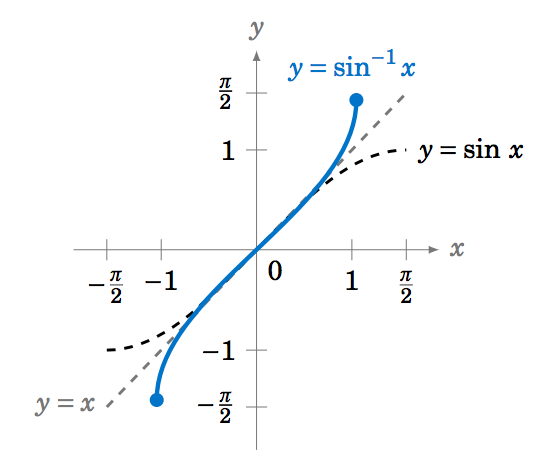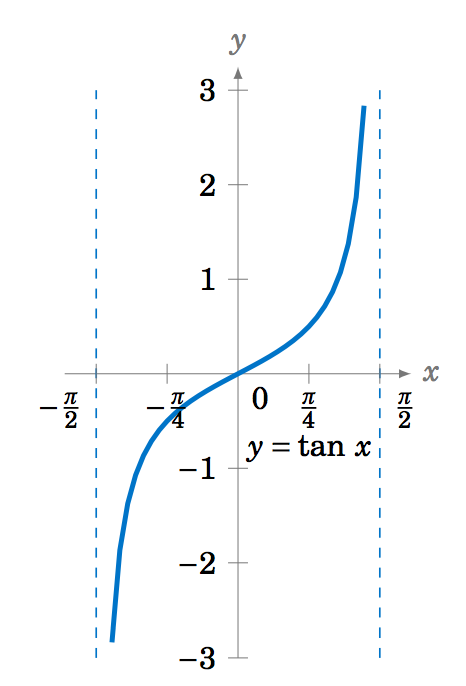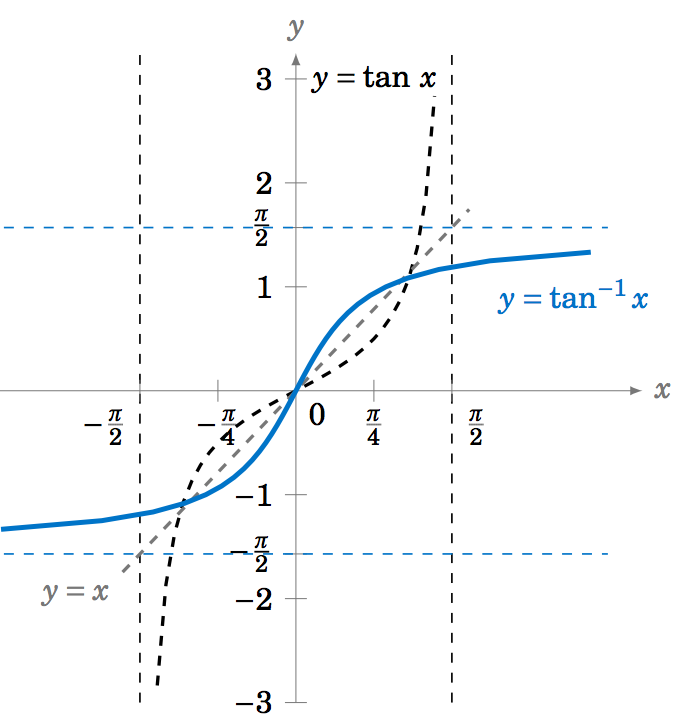$$\newcommand{\id}{\mathrm{id}}$$ $$\newcommand{\Span}{\mathrm{span}}$$ $$\newcommand{\kernel}{\mathrm{null}\,}$$ $$\newcommand{\range}{\mathrm{range}\,}$$ $$\newcommand{\RealPart}{\mathrm{Re}}$$ $$\newcommand{\ImaginaryPart}{\mathrm{Im}}$$ $$\newcommand{\Argument}{\mathrm{Arg}}$$ $$\newcommand{\norm}{\| #1 \|}$$ $$\newcommand{\inner}{\langle #1, #2 \rangle}$$ $$\newcommand{\Span}{\mathrm{span}}$$

# 1.8: Limits and continuity of Inverse Trigonometric functions

$$\newcommand{\vecs}{\overset { \rightharpoonup} {\mathbf{#1}} }$$ $$\newcommand{\vecd}{\overset{-\!-\!\rightharpoonup}{\vphantom{a}\smash {#1}}}$$$$\newcommand{\id}{\mathrm{id}}$$ $$\newcommand{\Span}{\mathrm{span}}$$ $$\newcommand{\kernel}{\mathrm{null}\,}$$ $$\newcommand{\range}{\mathrm{range}\,}$$ $$\newcommand{\RealPart}{\mathrm{Re}}$$ $$\newcommand{\ImaginaryPart}{\mathrm{Im}}$$ $$\newcommand{\Argument}{\mathrm{Arg}}$$ $$\newcommand{\norm}{\| #1 \|}$$ $$\newcommand{\inner}{\langle #1, #2 \rangle}$$ $$\newcommand{\Span}{\mathrm{span}}$$ $$\newcommand{\id}{\mathrm{id}}$$ $$\newcommand{\Span}{\mathrm{span}}$$ $$\newcommand{\kernel}{\mathrm{null}\,}$$ $$\newcommand{\range}{\mathrm{range}\,}$$ $$\newcommand{\RealPart}{\mathrm{Re}}$$ $$\newcommand{\ImaginaryPart}{\mathrm{Im}}$$ $$\newcommand{\Argument}{\mathrm{Arg}}$$ $$\newcommand{\norm}{\| #1 \|}$$ $$\newcommand{\inner}{\langle #1, #2 \rangle}$$ $$\newcommand{\Span}{\mathrm{span}}$$

## Inverse functions

Recall that a function $$f$$ is one-to-one (often written as $$1-1$$) if it assigns distinct values of $$y$$ to distinct values of $$x$$. In other words, if $$x_1 \ne x_2$$ then $$f(x_1 ) \ne f(x_2 )$$. Equivalently, $$f$$ is one-to-one if $$f(x_1 ) = f(x_2 )$$ implies $$x_1 = x_2$$. There is a simple horizontal rule for determining whether a function $$y=f(x)$$ is one-to-one: $$f$$ is one-to-one if and only if every horizontal line intersects the graph of $$y=f(x)$$ in the $$xy$$-coordinate plane at most once (see Figure 5.3.3).Figure 5.3.3 Horizontal rule for one-to-one functions

If a function $$f$$ is one-to-one on its domain, then $$f$$ has an inverse function, denoted by $$f^{-1}$$, such that $$y=f(x)$$ if and only if $$f^{-1}(y) = x$$. The domain of $$f^{-1}$$ is the range of $$f$$.

The basic idea is that $$f^{-1}$$ "undoes'' what $$f$$ does, and vice versa. In other words,
\nonumber \begin{alignat*}{3} f^{-1}(f(x)) ~&=~ x \quad&&\text{for all $$x$$ in the domain of $$f$$, and}\\ \nonumber f(f^{-1}(y)) ~&=~ y \quad&&\text{for all $$y$$ in the range of $$f$$.} \end{alignat*}

Theorem $$\PageIndex{1}$$

If $$f$$ is continuous and one to one, then $$f^{-1}\ is continuous on its domain. ## Inverse Trigonometric functions We know from their graphs that none of the trigonometric functions are one-to-one over their entire domains. However, we can restrict those functions to subsets of their domains where they are one-to-one. For example, \(y=\sin\;x$$ is one-to-one over the interval $$\left[ -\frac{\pi}{2},\frac{\pi}{2} \right]$$, as we see in the graph below:For $$-\frac{\pi}{2} \le x \le \frac{\pi}{2}$$ we have $$-1 \le \sin\;x \le 1$$, so we can define the inverse sine function $$y=\sin^{-1} x$$ (sometimes called the arc sine and denoted by $$y=\arcsin\;(x$$) whose domain is the interval $$[-1,1]$$ and whose range is the interval $$\left[ -\frac{\pi}{2},\frac{\pi}{2} \right]$$. In other words:

\begin{alignat}{3} \sin^{-1} (\sin\;y) ~&=~ y \quad&&\text{for $$-\tfrac{\pi}{2} \le y \le \tfrac{\pi}{2}$$}\label{eqn:arcsin1}\\ \sin\;(\sin^{-1} x) ~&=~ x \quad&&\text{for $$-1 \le x \le 1$$}\label{eqn:arcsin2} \end{alignat}

## Summary of Inverse Trigonometric functions

Lets illustrate the summary of Trigonometric functions and Inverse Trigonometric functions in following table:

 Trigonometric function graph of the Trigonometric function Restricted domain and the range Inverse Trigonometric function graph of the Inverse Trigonometric function Properties $$f(x)=\sin(x)$$$$\left[ -\frac{\pi}{2},\frac{\pi}{2} \right]$$ and $$[-1,1]$$ $$f^{-1}(x)=\sin^{-1} x$$$$f(x)=\cos(x)$$$$[0,\pi]$$ and $$[-1,1]$$ $$f^{-1}(x)=\cos^{-1} x$$\begin{alignat}{3} \cos^{-1} (\cos\;y) ~&=~ y \quad&&\text{for $$0 \le y \le \pi$$}\label{eqn:arccos1}\\ \cos\;(\cos^{-1} x) ~&=~ x \quad&&\text{for $$-1 \le x \le 1$$}\label{eqn:arccos2} \end{alignat} $$f(x)=\tan(x)$$$$\left( -\frac{\pi}{2},\frac{\pi}{2} \right)$$ and $$\mathbb{R}$$ $$f^{-1}(x)=\tan^{-1} x$$\begin{alignat}{3} \tan^{-1} (\tan\;y) ~&=~ y \quad&&\text{for $$-\tfrac{\pi}{2} < y < \tfrac{\pi}{2}$$}\label{eqn:arctan1}\\ \tan\;(\tan^{-1} x) ~&=~ x \quad&&\text{for all real $$x$$}\label{eqn:arctan2} \end{alignat} $$f(x)=\cot(x)$$\begin{alignat}{3} \cot^{-1} (\cot\;y) ~&=~ y \quad&&\text{for $$0 < y < \pi$$}\label{eqn:arccot1}\\ \cot\;(\cot^{-1} x) ~&=~ x \quad&&\text{for all real $$x$$}\label{eqn:arccot2} \end{alignat} $$f(x)=\sec(x)$$ $$[0,\pi]$$, with $$x\ne \frac{\pi}{2}$$ and $$\mathbb{R}$$\begin{alignat}{3} \csc^{-1} (\csc\;y) ~&=~ y \quad&&\text{for $$-\frac{\pi}{2} \le y \le \frac{\pi}{2}$$, $$y \ne 0$$}\label{eqn:arccsc1}\\ \csc\;(\csc^{-1} x) ~&=~ x \quad&&\text{for $$|x| \ge 1$$}\label{eqn:arccsc2} \end{alignat} \begin{alignat}{3} \sec^{-1} (\sec\;y) ~&=~ y \quad&&\text{for $$0 \le y \le \pi$$, $$y \ne \frac{\pi}{2}$$}\label{eqn:arcsec1}\\ \sec\;(\sec^{-1} x) ~&=~ x \quad&&\text{for $$|x| \ge 1$$}\label{eqn:arcsec2} \end{alignat}

Below are examples:

Example $$\PageIndex{1}$$:

Find $$\sin^{-1} \left(\sin\;\frac{\pi}{4}\right)$$.

Solution

Since $$-\frac{\pi}{2} \le \frac{\pi}{4} \le \frac{\pi}{2}$$, we know that $$\sin^{-1} \left(\sin\;\frac{\pi}{4}\right) = \boxed{\frac{\pi}{4}}\;$$, by Equation \ref{eqn:arcsin1}.

Example $$\PageIndex{2}$$:

Find $$\sin^{-1} \left(\sin\;\frac{5\pi}{4}\right)$$.

Solution

Since $$\frac{5\pi}{4} > \frac{\pi}{2}$$, we can not use Equation \ref{eqn:arcsin1}. But we know that $$\sin\;\frac{5\pi}{4} = -\frac{1}{\sqrt{2}}$$. Thus, $$\sin^{-1} \left(\sin\;\frac{5\pi}{4}\right) = \sin^{-1} \left( -\frac{1}{\sqrt{2}} \right)$$ is, by definition, the angle $$y$$ such that $$-\frac{\pi}{2} \le y \le \frac{\pi}{2}$$ and $$\sin\;y = -\frac{1}{\sqrt{2}}$$. That angle is $$y=-\frac{\pi}{4}$$, since

$\sin\;\left( -\tfrac{\pi}{4} \right) ~=~ -\sin\;\left( \tfrac{\pi}{4} \right) ~=~ -\tfrac{1}{\sqrt{2}} ~. \nonumber$

Example $$\PageIndex{3}$$:

Find $$\cos^{-1} \left(\cos\;\frac{\pi}{3}\right)$$.

Solution

Since $$0 \le \frac{\pi}{3} \le \pi$$, we know that $$\cos^{-1} \left(\cos\;\frac{\pi}{3}\right) = \boxed{\frac{\pi}{3}}\;$$, by Equation \ref{eqn:arccos1}.

Example $$\PageIndex{4}$$:

Find $$\cos^{-1} \left(\cos\;\frac{4\pi}{3}\right)$$.

Solution

Since $$\frac{4\pi}{3} > \pi$$, we can not use Equation \ref{eqn:arccos1}. But we know that $$\cos\;\frac{4\pi}{3} = -\frac{1}{2}$$. Thus, $$\cos^{-1} \left(\cos\;\frac{4\pi}{3}\right) = \cos^{-1} \left( -\frac{1}{2} \right)$$ is, by definition, the angle $$y$$ such that $$0 \le y \le \pi$$ and $$\cos\;y = -\frac{1}{2}$$. That angle is $$y=\frac{2\pi}{3}$$ (i.e. $$120^\circ$$). Thus, $$\cos^{-1} \left(\cos\;\frac{4\pi}{3}\right) = \boxed{\tfrac{2\pi}{3}}\;$$.

Example $$\PageIndex{5}$$:

Find $$\tan^{-1} \left(\tan\;\frac{\pi}{4}\right)$$.

Solution

Since $$-\tfrac{\pi}{2} \le \tfrac{\pi}{4} \le \tfrac{\pi}{2}$$, we know that $$\tan^{-1} \left(\tan\;\frac{\pi}{4}\right) = \boxed{\frac{\pi}{4}}\;$$, by Equation \ref{eqn:arctan1}.

Example $$\PageIndex{6}$$:

Find $$\tan^{-1} \left(\tan\;\pi\right)$$.

Solution

Since $$\pi > \tfrac{\pi}{2}$$, we can not use Equation \ref{eqn:arctan1}. But we know that $$\tan\;\pi = 0$$. Thus, $$\tan^{-1} \left(\tan\;\pi\right) = \tan^{-1} 0$$ is, by definition, the angle $$y$$ such that $$-\tfrac{\pi}{2} \le y \le \tfrac{\pi}{2}$$ and $$\tan\;y = 0$$. That angle is $$y=0$$. Thus, $$\tan^{-1} \left(\tan\;\pi \right) = \boxed{0}\;$$.

Example$$\PageIndex{7}$$:

Find the exact value of $$\cos\;\left(\sin^{-1}\;\left(-\frac{1}{4}\right)\right)$$.

Solution

Let $$\theta = \sin^{-1}\;\left(-\frac{1}{4}\right)$$. We know that $$-\tfrac{\pi}{2} \le \theta \le \tfrac{\pi}{2}$$, so since $$\sin\;\theta = -\frac{1}{4} < 0$$, $$\theta$$ must be in QIV. Hence $$\cos\;\theta > 0$$. Thus,

$\cos^2 \;\theta ~=~ 1 ~-~ \sin^2 \;\theta ~=~ 1 ~-~ \left( -\frac{1}{4} \right)^2 ~=~\frac{15}{16} \quad\Rightarrow\quad \cos\;\theta ~=~ \frac{\sqrt{15}}{4} ~. \nonumber$

Note that we took the positive square root above since $$\cos\;\theta > 0$$. Thus, $$\cos\;\left(\sin^{-1}\;\left(-\frac{1}{4}\right)\right) = \boxed{\frac{\sqrt{15}}{4}}\;$$.

Example $$\PageIndex{8}$$:

Show that $$\tan\;(\sin^{-1} x) = \dfrac{x}{\sqrt{1 - x^2}}$$ for $$-1 < x < 1$$.

Solution

When $$x=0$$, the Equation holds trivially, since

$\nonumber \tan\;(\sin^{-1} 0) ~=~ \tan\;0 ~=~ 0 ~=~ \dfrac{0}{\sqrt{1 - 0^2}} ~.$

Now suppose that $$0 < x < 1$$. Let $$\theta = \sin^{-1} x$$. Then $$\theta$$ is in QI and $$\sin\;\theta = x$$. Draw a right triangle with an angle $$\theta$$ such that the opposite leg has length $$x$$ and the hypotenuse has length $$1$$, as in Figure 5.3.10 (note that this is possible since $$0 < x < 1$$). Then $$\sin\;\theta = \frac{x}{1} = x$$. By the Pythagorean Theorem, the adjacent leg has length $$\sqrt{1 - x^2}$$. Thus, $$\tan\;\theta = \frac{x}{\sqrt{1 - x^2}}$$.

If $$-1 < x < 0$$ then $$\theta = \sin^{-1} x$$ is in QIV. So we can draw the same triangle except that it would be "upside down'' and we would again have $$\tan\;\theta = \frac{x}{\sqrt{1 - x^2}}$$, since the tangent and sine have the same sign (negative) in QIV. Thus, $$\tan\;(\sin^{-1} x) = \dfrac{x}{\sqrt{1 - x^2}}$$ for $$-1 < x < 1$$.Example $$\PageIndex{9}$$:

Prove the identity $$\tan^{-1} x \;+\; \cot^{-1} x ~=~ \frac{\pi}{2}$$.

Solution:

Let $$\theta = \cot^{-1} x$$. Using relations, we have

$\nonumber \tan\;\left( \tfrac{\pi}{2} - \theta \right) ~=~ -\tan\;\left( \theta - \tfrac{\pi}{2} \right) ~=~ \cot\;\theta ~=~ \cot\;(\cot^{-1} x) ~=~ x ~,$

by Equation \ref{eqn:arccot2}. So since $$\tan\;(\tan^{-1} x) = x$$ for all $$x$$, this means that $$\tan\;(\tan^{-1} x) = \tan\;\left( \tfrac{\pi}{2} - \theta \right)$$. Thus, $$\tan\;(\tan^{-1} x) = \tan\;\left( \tfrac{\pi}{2} - \cot^{-1} x \right)$$. Now, we know that $$0 < \cot^{-1} x < \pi$$, so $$-\tfrac{\pi}{2} < \tfrac{\pi}{2} - \cot^{-1} x < \tfrac{\pi}{2}$$, i.e. $$\tfrac{\pi}{2} - \cot^{-1} x$$ is in the restricted subset on which the tangent function is one-to-one. Hence, $$\tan\;(\tan^{-1} x) = \tan\;\left( \tfrac{\pi}{2} - \cot^{-1} x \right)$$ implies that $$\tan^{-1} x = \tfrac{\pi}{2} - \cot^{-1} x$$, which proves the identity.

## Continuity of Inverse Trigonometric functions

Example $$\PageIndex{1}$$:

Let $$f(x)= \frac{3 \sec^{-1} (x)}{4-\tan^{-1}( x)}$$. Find the values(if any) for which $$f(x)$$ is continuous.

Exercise $$\PageIndex{1}$$

Let $$f(x)= \frac{3 \sec^{-1} (x)}{8+2\tan^{-1}( x)}$$. Find the values(if any) for which $$f(x)$$ is continuous.

## Limit of Inverse Trigonometric functions

Theorem $$\PageIndex{1}$$

$$lim_{x \rightarrow \infty} \tan^{-1}( x) = \frac{\pi}{2}$$.

$$lim_{x \rightarrow -\infty} \tan^{-1}( x) = -\frac{\pi}{2}$$.

$$lim_{x \rightarrow \infty} \sec^{-1} (x)=\lim _{x \rightarrow \infty} \sec^{-1} (x )= \frac{\pi}{2}$$.

Example $$\PageIndex{1}$$:

Find $$\lim_{x \rightarrow \infty} sin\left( 2\tan^{-1}( x)\right)$$.

Exercise $$\PageIndex{1}$$

Find $$\lim_{x \rightarrow -\infty} sin\left( 2\tan^{-1}( x)\right)$$.